Specific gravity test of bitumen

A specific gravity test measures how much lighter or heavier bitumen is compared with the same volume of water. There are two methods to calculate the specific gravity of bitumen, the Pycnometer method, and the Balance method.

The specific gravity of pure bitumen is in the range of 0.97 to 1.02.

When the specific gravity is less than 1 it shows the bitumen is lighter than water and when the value is more than 1 it indicates the bitumen is heavier than water.

Bitumen tends to float on the water surface more when the value is less than 1. also it tends to settle down when the value is more than 1.

Following is a table of specific gravities for different penetration grades of bitumen based on ASTM D70:

 Penetration grade bitumen Specific gravity @ 25°C min max 30/40 1.01 1.05 40/50 1.01 1.05 60/70 1.01 1.06 85/100 1.01 1.05 100/120 1.01 1.04

Why is specific gravity test of bitumen important?

A specific gravity test can be used to classify bitumen. With help of it, we can identify the type of bitumen. For example, bitumen 80/100 has a different specific gravity value from bitumen 30/40.

Also to control the quality of bitumen we use its specific gravity. By using that, the impurity of bitumen is recognizable. Because impure bitumen has higher specific gravity.

For road construction, bitumen is used with aggregates. To have a suitable and stable asphalt, the optimal volume of bitumen must be used. Because too much bitumen makes asphalt sticky. In addition, when there isn’t enough bitumen, the aggregates don’t stick together.

The bitumen binder should coat the space between the aggregates well. For this reason, we need to calculate the density of voids.

The volume of voids is determined by the density of bitumen and the density of aggregates.

These calculations are performed using Marshal Mix Design.

The volume of bitumen is necessary for Marshal Mix Design. However, the volume is converted to mass when we mix the bitumen and aggregates. Because measuring weight is easier than measuring the volume.

Weight = Specific Gravity * Volume

International standard methods for specific gravity test of bitumen

Various standard methods are provided to determine the specific gravity of bitumen, as follows:

• ASTM D 70
• AASHTO T 228
• IS 1202
• EN 15326
• IP 549

The component of the apparatus and test steps of specific gravity:

In the following we describe the procedure of calculating specific gravity based on two methods:

1. Pycnometer method ( ASTM D70 )
2. Balance method ( IS 1202

1. Pycnometer method

• Apparatus

1. Specific gravity bottle (pycnometer):  The ordinary specific gravity bottle shall be used for materials that remain fluid at 25 °C. On the other hand, the wide mouth capillary type shall be used for materials that remain semi-solid or high viscous at 25 °C.
2. Oven
3. Heater
4. Water bath
5. Thermometer
6. Electronic balance(a)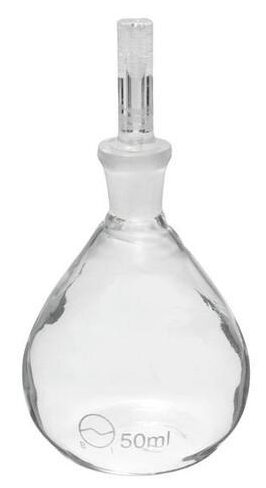(b)

Figure1 : (a) Wide mouth capillary specific gravity bottle. (b) Ordinary specific gravity bottle.

• Procedure

Wash the specific gravity bottle (pycnometer) and place it in the oven to dry. Weigh it with its stopper. Consider the value as A.

Fill the pycnometer with distilled water and insert the stopper. Put it in a water bath at 25 °C degrees and wait for it to warm. Then take the pycnometer out of the water bath and dry it with a towel. Record the weight with the balance and consider it as B.

Heat the bitumen between 75 °C and 100 °C to become completely liquid. Fill half of a clean and dry pycnometer with bitumen. Be careful that air does not enter the sample.

Allow it to cool at room temperature. Then place in a water bath at a temperature of 25 °C to reach the bath temperature. Dry it completely then weigh it and consider the value as C.

At the next step, fill the other half of the bottle with distilled water. Place it in a water bath at a temperature of 25 °C to be at the same time as the water bath. Dry it and weigh it. Consider the value as D.

Finally, the mass of the pycnometer which is filled with bitumen is measured. Consider that value as E.

Use the following formula to calculate the specific gravity:

For solid and semi-solid bitumen:

Specific gravity= (C-A)/[(B-A)-(D-C)]

For liquid bitumen:

Specific gravity=(E-A)/(B-A)

Table 1: A summary of the values measured during the test

 A= mass of pycnometer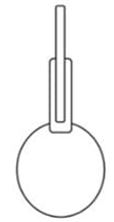B= mass of the pycnometer is filled with water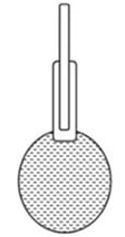C= mass of pycnometer about half-filled with the bitumen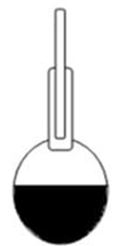D = mass of the pycnometer about half-filled with the material and the rest with distilled water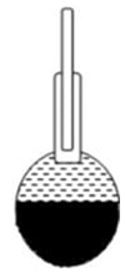E = mass of the pycnometer filled with the bitumen.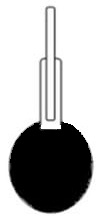2. Balance method

• Apparatus

1. Balance straddle: It has a pan straddle with a suitable size to support a beaker. It should be suitable to determine the weight of the specimen in water.
2. Analytical balance scale
3. Thermometer
5. Cubic brass mould• Sample preparation:

Heat the bitumen sample gently. Coat the cubic mould brass with a mixture of glycerine and dextrin.

Pour the specimen into the mould and fill it. It is better to fill it in a little more than needed. Then cut the excess amount with a hot knife.

Let the specimen cool at room temperature. After that remove it from the brass mould.

• Test:

Hang a piece of wax silk thread from the balance and tie it. The length of the thread should be long enough to reach the pan.

Attach the sample to the thread and weigh it.

As the sample is suspended from the thread, place it in a water bath at 25 °C and weigh it.

Calculate the specific gravity of the material as

follows:

Specific gravity = A/(A-B)

A = mass of the dry specimen

B = mass of the specimen when immersed in distilled water.